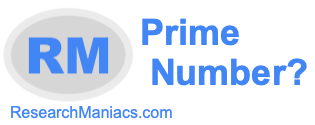Is 31 a prime number?To find out if 31 is a prime number, we went through our prime number check list. Everything on our prime number check list must be true for 31 to be a prime number.

For 31 to be a prime number, it must be an integer and a positive number: True

For 31 to be a prime number, it must be a number greater than 1: True

For 31 to be a prime number, it must be the number 2 or an odd number greater than 2: True

For 31 to be a prime number, it must only be divisible by 1 and itself: True

All of our tests above proved true, therefore the answer to the question: "Is 31 a prime number?" is as follows:

YES: 31 is a prime number.

What is a prime number?
Enter another number below to see if it is a prime number.

Is 32 a prime number?
Using the knowledge you learned above to figure out if 31 is a prime number, do you know the answer the next number on our list?